Home > A2C > Chapter 8 > Lesson 8.1.4 > Problem8-54

8-54.
1. The measure of ∠ROS in ΔROS below is 60°. Homework Help ✎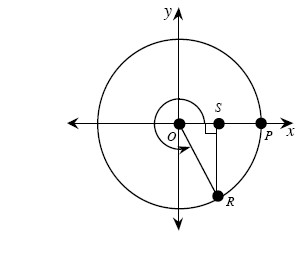1. The curved arrow represents the rotation of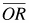, beginning from the positive x-axis. Through how many degrees hasrotated?

2. If OR = 1, what are the exact length of OS and SR?

3. What are the exact coordinates of point R?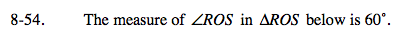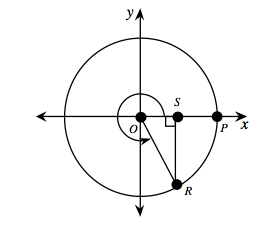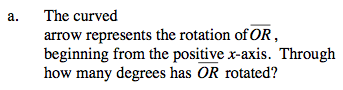Remember that a whole circle is 360°.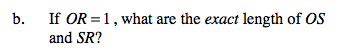Remember the 30°-60°-90° triangle.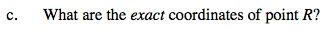$\text{(cos(300\degree), sin(300\degree)) or \left(\frac{1}{2},-\frac{\sqrt{3}}{2}\right)}$